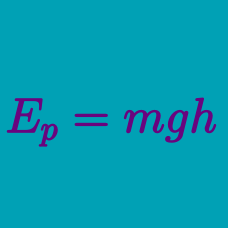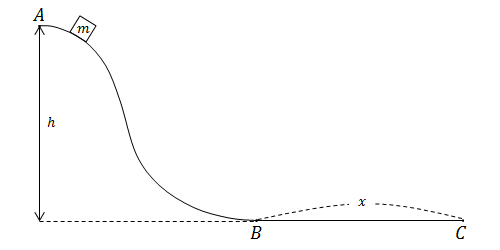Classical Mechanics

# Potential Energy - problem solvingA grandfather clock works by a pendulum system and keeps time because the pendulum has a constant frequency. The grandfather clock in my room has stopped, but I'm too lazy to get out of bed to start the pendulum oscillating again. Hence I throw a sticky piece of gum at the pendulum to get it moving again. My piece of gum has a mass of 10 g, hits the pendulum inelastically with a speed of 10 m/s, and sticks there. Admittedly disgusting. But, more importantly, if the pendulum has a mass of 90 g, how high does the pendulum on my grandfather clock go in meters?

Details and assumptions

• The acceleration due to gravity is $9.8~m/s^2$.
• Treat the grandfather clock as a simple pendulum.An object of mass $m=9\text{ kg}$ is released from rest at point $A,$ and slides down a long, frictionless, $h=60\text{ m}$ high slide. Then it enters the horizontal surface from point $B$ to $C,$ which is not frictionless, and comes to a complete stop at point $C.$ If the coefficient of kinetic friction between the object and the surface is $\mu=0.1,$ what is the horizontal distance $x$ between points $B$ and $C?$

The gravitational acceleration is $g=10\text{ m/s}^2.$

An object of mass $4\text{ kg}$ initially at rest falls freely towards a huge spring that is $100\text{ m}$ below. If the spring constant is $k=16\text{ N/m},$ what is the maximum change in the spring's length?

Air resistance is negligible and gravitational acceleration is $g=10\text{ m/s}^2.$

While an object of mass $10\text{ kg}$ drops from the edge of a $60$-meter-high cliff, it experiences air resistance, whose strength during the descent is constantly $15\text{ N}.$ What is the square of the velocity with which the object hits the ground?

A meteor of mass $m=800\text{ kg}$ enters the earth's atmosphere with a velocity of $500\text{ m/s},$ and falls vertically toward the ground. The meteor burns while falling, and its mass decreases at a rate of $5\text{ kg/s}.$

If the meteor falls with a constant velocity, how much gravitational potential energy will it lose in $16$ seconds?

Note

• The height of the meteor when it enters the atmosphere is $1000\text{ km},$
• The gravitational acceleration is $g=10\text{ m/s}^2.$
×